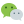# 函数申明

``````function fn() {
console.log('run');
}

fn();  // run
``````

``````fn();

function fn() {
console.log('run');
}
// run
``````

``````fn();

var fn = function() {
console.log('run');
}
// TypeError: fn is not a function
``````

# 变量申明

``````var a = 1;
console.log(a);
// 1
``````

``````console.log(a);
var a = 1;
``````

``````undefined
``````

``````var a;
console.log(a);
// undefined
``````

``````fn();

var fn = function() {
console.log('run');
}
// TypeError: fn is not a function
``````

# 拓展

## return 的限制

``````function fn() {
console.log(a);
fnn();
return ;

var a = 1;
function fnn() {
console.log('exist.')
}
}

fn();
// undefined
// exist.
``````

## 提升优先级

``````function fn1() {
console.log(a);
var a = 1;
function a(){};
}
function fn2() {
console.log(a);
function a(){};
var a = 1;
}

fn1(); // f a() {}
fn2(); // f a() {}
``````

## 提升的执行

``````function fn() {
fnn();

var a = 1;
function fnn() {
console.log(a);
}
}

fn(); // undefined
``````

1. 执行 fn();
2. 执行 fnn();
1. 发现前面没有关于函数 fnn() 的申明，于是向后寻找，最后找到了；
2. 执行 console.log(a);
3. 发现 fnn 内部没有 a 的定义，向外一层寻找；
3. a 申明在 fnn() 执行语句以后，所以 a 触发提升，供之前的 console.log 使用；

``````function fn() {
console.log(a);
var a = 1;
}

fn();
``````

# var, let, const的区别

JavaScript 中申明变量的方式以及对应效果如下：

``````a = 0;       // 全局变量
var b = 1;   // 局部作用域变量（当前上下文）
let c = 2;   // 块级作用域变量（当前块级上下文）
const d = 3; // 常量
``````

## 作用域

``````var a = '全局';
function fn() {
var aa = '局部'
console.log(aa);
}
if (true) {
var b = '全局';
let bb = '块级';
const bbb = '块级';
}
for (i = 0; i < 1; i++) {
var c = '全局'
let cc = '块级';
const ccc = '块级';
}

console.log(a);  // “全局”
fn();  // “局部”
console.log(aa); // aa is not defined
console.log(b, c); // “全局” “全局”
console.log(bb, cc); // bb is not defined  cc is not defined
console.log(bbb, ccc); // bbb is not defined  ccc is not defined
``````

## 全局变量提升

``````console.log(a);
a = 0;
// ReferenceError: a is not defined
``````

``````console.log(a); // a 前面未申明
// ReferenceError: a is not defined
``````

## 局部变量提升

``````function fn() {
console.log(aa);
var aa = 1;
}
console.log(a);
var a = 1;
// undefined
fn();
// undefined
``````

``````if (false) {
var a = 1;
}
console.log(a);
// undefined
``````

## 块级变量提升

``````if (true) {
console.log(a);
let a = 1;
console.log(aa);
}
// ReferenceError: Cannot access 'a' before initialization
// ReferenceError: aa is not defined

if (true) {
console.log(b);
const b = 1;
console.log(bb);
}
// ReferenceError: Cannot access 'b' before initialization
// ReferenceError: bb is not defined
``````

### 评论：微信公众号：程序骑士